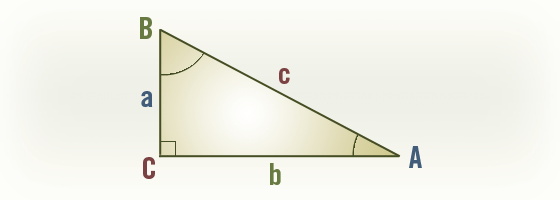# Revit Families 104 – Trigonometry for Right Triangles

• Posted on April 14, 2011

Prior to reading this post we recommend reading “Revit Families 103 – Formula Basics” to get the complete overview of all the basic operations allowed in Revit formulas.

Many times when working with Revit Formula in family creation you will want to work with right triangles. The following is meant as a handy reference for you to make working with right triangles a little easier.Known: a, b   c = sqrt(a ^ 2 + b ^ 2) A = atan(a / b) B = atan(b / a) Known: a, c b = sqrt(c ^ 2 – a ^ 2) A = asin(a / c) B = acos(a / c) Known: b, c a = sqrt(c ^ 2 – b ^ 2) A = acos(b / c) B = asin(b / c) Known: c, A a = c * sin(A) b = c * cos(A) B = 90° – A Known: c, B a = c * cos(B) b = c * sin(B) A = 90° – B Known: a, B   b = a * tan(B) c = a / cos(B) A = 90° – B Known: b, A a = b * tan(A) c = b / cos(A) B = 90° – A Known: a, A b = a / tan(A) c = a / sin(A) B = 90° – A Known: b, B a = b / tan(B) c = b / sin(B) A = 90° – B